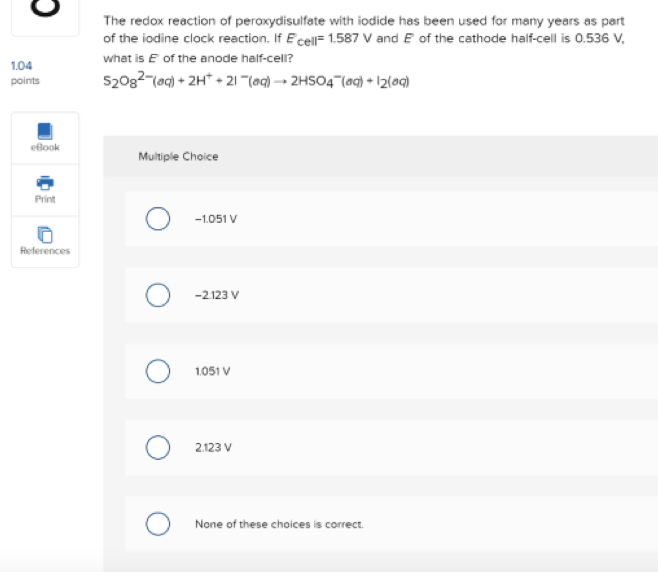# A 0.570 g sample of KCI is added to 39.9 g of water in a calorimeter. If the temperature decreases by 1.14°C, what is the approximate amount of heat (in 3) involved in the dissolution of the KCI, assuming the heat capacity of the resulting solution is 4.18 J/g°C? Is the reaction exothermic or endothermic? t exothermic endothermic